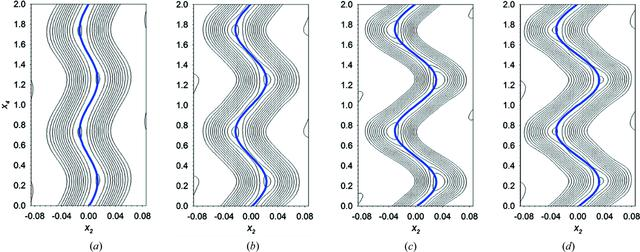disable zoom     view article Figure 4 (a)–(d) Contour plot of the x4-x2 two-dimensional sections calculated fixing x1 = 0.5 and x3 = 0, corresponding to the atomic domains of the cobalt atom, with x1, x2 and x3 corresponding with x, y and z axes, respectively, and x4 being the parameter of modulation t. The cobalt contour plots obtained at 86, 90, 106 and 122 K together with the refined modulation function, denoted as a solid blue line, are represented from (a) to (d), respectively.

IUCrJ
Volume 6| Part 1| January 2019| Pages 105-115
ISSN: 2052-2525